set.seed(12345) # for reproducibility
options(knitr.kable.NA = '')

# Some packages need to be loaded. We use pacman as a package manager, which takes care of the other packages.
if (!require("pacman", quietly = TRUE)) install.packages("pacman")
if (!require("Rmisc", quietly = TRUE)) install.packages("Rmisc") # Never load it directly.
pacman::p_load(tidyverse, knitr, car, afex, emmeans, parallel, ordinal,
ggbeeswarm, RVAideMemoire)

klippy::klippy()

# 1 Item Repetition Phase

Forty eight participants were recruited.1 Half of the participants were exposed to 24 famous faces while the other half were exposed to 24 non-famous faces (pre-experimental stimulus familiarity as a between-participants factor). Each face was repeated eight times, resulting in 192 trials in total. Participants made a male/female judgment for each face.

P1 <- read.csv("data/data_FamSM_Exp4A_Face_REP.csv", header = T)
P1$Familiarity = factor(P1$Familiarity, levels=c(1,2), labels=c("Famous","Non-famous"))

glimpse(P1, width=70)
## Observations: 9,216
## Variables: 9
## $SID <int> 1, 1, 1, 1, 1, 1, 1, 1, 1, 1, 1, 1, 1, 1, 1, 1,… ##$ Familiarity <fct> Non-famous, Non-famous, Non-famous, Non-famous,…
## $RepTime <int> 1, 1, 1, 1, 1, 1, 1, 1, 1, 1, 1, 1, 1, 1, 1, 1,… ##$ Trial       <int> 1, 2, 3, 4, 5, 6, 7, 8, 9, 10, 11, 12, 13, 14, …
## $ImgCat <int> 2, 1, 2, 1, 1, 2, 2, 2, 1, 2, 2, 1, 2, 1, 1, 1,… ##$ Resp        <int> 2, 1, 2, 1, 1, 2, 2, 1, 1, 2, 2, 1, 2, 1, 1, 1,…
## $Corr <int> 1, 1, 1, 1, 1, 1, 1, 0, 1, 1, 1, 1, 1, 1, 1, 1,… ##$ RT          <dbl> 609.87, 585.12, 481.17, 537.09, 521.08, 833.08,…
## $ImgName <fct> unknown_female06.jpg, unknown_male12.jpg, unkno… # 1. SID: participant ID # 2. Familiarity: pre-experimental familiarity. 1 = famous, 2 = non-famous # 3. RepTime: number of repetitions, 1~8 # 4. Trial: 1~24 # 5. ImgCat: stimulus category. male vs. female # 6. Resp: male/female judgment, 1 = male, 2 = female, 0 = no response # 7. Corr: correctness, 1=correct, 0 = incorrect or no response # 8. RT: reaction times in ms. # 9. ImgName: name of stimuli table(P1$Familiarity, P1$SID) ## ## 1 2 3 4 5 6 7 8 9 10 11 12 13 14 15 ## Famous 0 192 0 192 0 192 0 192 0 192 0 192 0 192 0 ## Non-famous 192 0 192 0 192 0 192 0 192 0 192 0 192 0 192 ## ## 16 17 18 19 20 21 22 23 24 25 26 27 28 29 30 ## Famous 192 0 192 0 192 0 192 0 192 0 192 0 192 0 192 ## Non-famous 0 192 0 192 0 192 0 192 0 192 0 192 0 192 0 ## ## 31 32 33 34 35 36 37 38 39 40 41 42 43 44 45 ## Famous 0 192 0 192 0 192 0 192 0 192 0 192 0 192 0 ## Non-famous 192 0 192 0 192 0 192 0 192 0 192 0 192 0 192 ## ## 46 47 48 ## Famous 192 0 192 ## Non-famous 0 192 0 ## 1.1 Accuracy We calculated the mean and s.d. of individual participants’ mean percentage accuracy. Overall accuracy of the male/female judgment was high. The famous condition showed lower mean accuracy and larger variance than the non-famous condition. In the following plot, red points and error bars represent the means and 95% CIs (error bars are hidden behind the points). # phase 1, subject-level, long-format P1slong <- P1 %>% group_by(SID, Familiarity) %>% summarise(Accuracy = mean(Corr)*100) %>% ungroup() # summary table P1slong %>% group_by(Familiarity) %>% summarise(M = mean(Accuracy), SD = sd(Accuracy)) %>% ungroup() %>% kable() Familiarity M SD Famous 97.80816 3.1286933 Non-famous 98.78472 0.8911482 # group level, needed for printing & geom_pointrange # Rmisc must be called indirectly due to incompatibility between plyr and dplyr. P1g <- Rmisc::summarySE(data = P1slong, measurevar = "Accuracy", groupvars = "Familiarity") ggplot(P1slong, aes(x=Familiarity, y=Accuracy)) + geom_violin(width = 0.5, trim=TRUE) + ggbeeswarm::geom_quasirandom(color = "blue", size = 3, alpha = 0.2, width = 0.2) + geom_pointrange(P1g, inherit.aes=FALSE, mapping=aes(x = Familiarity, y=Accuracy, ymin = Accuracy - ci, ymax = Accuracy + ci), colour="darkred", size = 1)+ coord_cartesian(ylim = c(50, 100), clip = "on") + labs(x = "Pre-experimental Stimulus Familiarity", y = "Male/Female Judgment \n Accuracy (%)") + theme_bw(base_size = 18)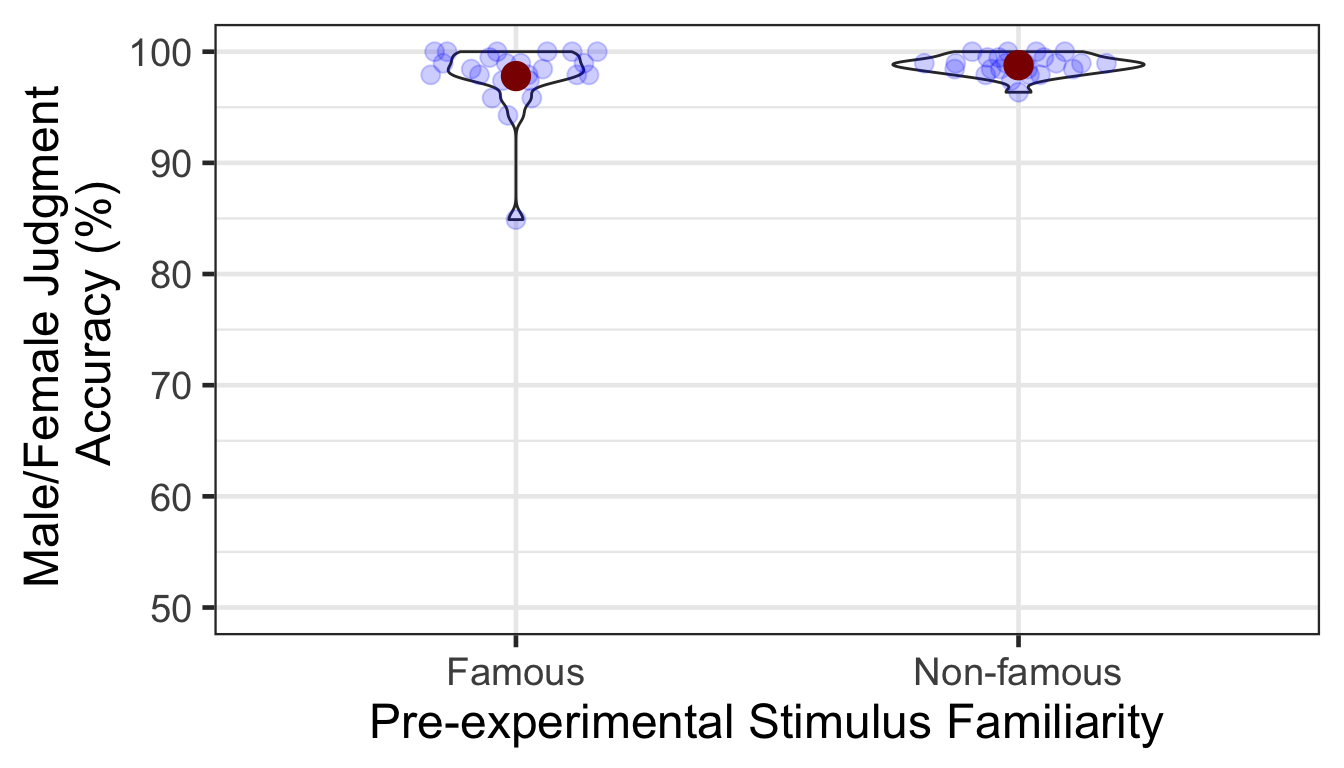### 1.1.1 ANOVA A one-way ANOVA showed that the accuracy was not significantly different between famous vs. non-famous conditions. p1.aov <- aov_ez(id = "SID", dv = "Accuracy", data = P1slong, between = "Familiarity") anova(p1.aov, es = "pes") %>% kable(digits = 4) num Df den Df MSE F pes Pr(>F) Familiarity 1 46 5.2914 2.1628 0.0449 0.1482 # 2 Item-Source Association Phase Participants learned 48 face-location (a quadrant on the screen) associations. They were instructed to pay attention to the location of each face while reporting in which quadrant a face appeared. A between-participants pre-experimental stimulus familiarity factor determined whether a participant viewed famous or non-famous faces. A within-participant item repetition factor determined whether a repeated item (which had been presented in the first phase) or an unrepeated item (which appeared for the first time in the item-source association phase) was presened in each trial. P2 <- read.csv("data/data_FamSM_Exp4A_Face_SRC.csv", header = T) P2$Familiarity = factor(P2$Familiarity, levels=c(1,2), labels=c("Famous","Non-famous")) P2$Repetition = factor(P2$Repetition, levels=c(1,2), labels=c("Repeated","Unrepeated")) glimpse(P2, width=70) ## Observations: 2,304 ## Variables: 10 ##$ SID         <int> 1, 1, 1, 1, 1, 1, 1, 1, 1, 1, 1, 1, 1, 1, 1, 1,…
## $Familiarity <fct> Non-famous, Non-famous, Non-famous, Non-famous,… ##$ Trial       <int> 1, 2, 3, 4, 5, 6, 7, 8, 9, 10, 11, 12, 13, 14, …
## $Repetition <fct> Repeated, Unrepeated, Repeated, Repeated, Unrep… ##$ ImgCat      <int> 2, 1, 1, 1, 1, 2, 2, 1, 1, 2, 1, 2, 1, 1, 1, 2,…
## $Loc <int> 4, 4, 1, 1, 4, 4, 2, 1, 4, 1, 3, 2, 3, 2, 4, 4,… ##$ Resp        <int> 4, 4, 1, 1, 4, 4, 2, 1, 4, 1, 3, 2, 3, 2, 4, 4,…
## $Corr <int> 1, 1, 1, 1, 1, 1, 1, 1, 1, 1, 1, 1, 1, 1, 1, 1,… ##$ RT          <dbl> 1127.69, 867.79, 879.91, 540.00, 840.05, 644.18…
## $ImgName <fct> unknown_female21.jpg, unknown_male18.jpg, unkno… # 1. SID: participant ID # 2. Familiarity: pre-experimental familiarity. 1 = famous, 2 = non-famous # 3. Trial: 1~48 # 4. Repetition: 1 = repeated, 2 = unrepeated # 5. ImgCat: stimulus category. male vs. female # 6. Loc: location (source) of memory item; quadrants, 1~4 # 7. Resp: male/female judgment, 1 = male, 2 = female, 0 = no response # 8. Corr: correctness, 1 = correct, 0 = incorrect or no response # 9. RT: reaction times in ms. # 10. ImgName: name of stimuli table(P2$Familiarity, P2$SID) ## ## 1 2 3 4 5 6 7 8 9 10 11 12 13 14 15 16 17 18 19 20 ## Famous 0 48 0 48 0 48 0 48 0 48 0 48 0 48 0 48 0 48 0 48 ## Non-famous 48 0 48 0 48 0 48 0 48 0 48 0 48 0 48 0 48 0 48 0 ## ## 21 22 23 24 25 26 27 28 29 30 31 32 33 34 35 36 37 38 39 40 ## Famous 0 48 0 48 0 48 0 48 0 48 0 48 0 48 0 48 0 48 0 48 ## Non-famous 48 0 48 0 48 0 48 0 48 0 48 0 48 0 48 0 48 0 48 0 ## ## 41 42 43 44 45 46 47 48 ## Famous 0 48 0 48 0 48 0 48 ## Non-famous 48 0 48 0 48 0 48 0 table(P2$Repetition, P2$SID) ## ## 1 2 3 4 5 6 7 8 9 10 11 12 13 14 15 16 17 18 19 20 ## Repeated 24 24 24 24 24 24 24 24 24 24 24 24 24 24 24 24 24 24 24 24 ## Unrepeated 24 24 24 24 24 24 24 24 24 24 24 24 24 24 24 24 24 24 24 24 ## ## 21 22 23 24 25 26 27 28 29 30 31 32 33 34 35 36 37 38 39 40 ## Repeated 24 24 24 24 24 24 24 24 24 24 24 24 24 24 24 24 24 24 24 24 ## Unrepeated 24 24 24 24 24 24 24 24 24 24 24 24 24 24 24 24 24 24 24 24 ## ## 41 42 43 44 45 46 47 48 ## Repeated 24 24 24 24 24 24 24 24 ## Unrepeated 24 24 24 24 24 24 24 24 ## 2.1 Accuracy We calculated the mean and s.d. of individual participants’ mean percentage accuracy. The overall performance was at ceiling. # phase 2, subject-level, long-format P2slong <- P2 %>% group_by(SID, Familiarity, Repetition) %>% summarise(Accuracy = mean(Corr)*100) %>% ungroup() # summary table P2g <- P2slong %>% group_by(Familiarity, Repetition) %>% summarise(M = mean(Accuracy), SD = sd(Accuracy)) %>% ungroup() P2g %>% kable() Familiarity Repetition M SD Famous Repeated 99.65278 1.1763744 Famous Unrepeated 99.13194 2.1207391 Non-famous Repeated 99.65278 1.1763744 Non-famous Unrepeated 99.82639 0.8505173 # group level, needed for printing & geom_pointrange # Rmisc must be called indirectly due to incompatibility between plyr and dplyr. P2g$ci <- Rmisc::summarySEwithin(data = P2slong, measurevar = "Accuracy", idvar = "SID",
withinvars = "Repetition", betweenvars = "Familiarity")$ci P2g$Accuracy <- P2g$M ggplot(data=P2slong, aes(x=Familiarity, y=Accuracy, fill=Repetition)) + geom_violin(width = 0.7, trim=TRUE) + ggbeeswarm::geom_quasirandom(dodge.width = 0.7, color = "blue", size = 3, alpha = 0.2, show.legend = FALSE) + # geom_pointrange(data=P2g, # aes(x = Familiarity, ymin = Accuracy-ci, ymax = Accuracy+ci, color = Repetition), # position = position_dodge(0.7), color = "darkred", size = 1, show.legend = FALSE) + coord_cartesian(ylim = c(50, 100), clip = "on") + labs(x = "Pre-experimental Stimulus Familiarity", y = "Localization Accuracy (%)", fill='Item Repetition') + scale_fill_manual(values=c("#E69F00", "#56B4E9"), labels=c("Repeated", "Unrepeated")) + theme_bw(base_size = 18) + theme(panel.grid.major = element_blank(), panel.grid.minor = element_blank())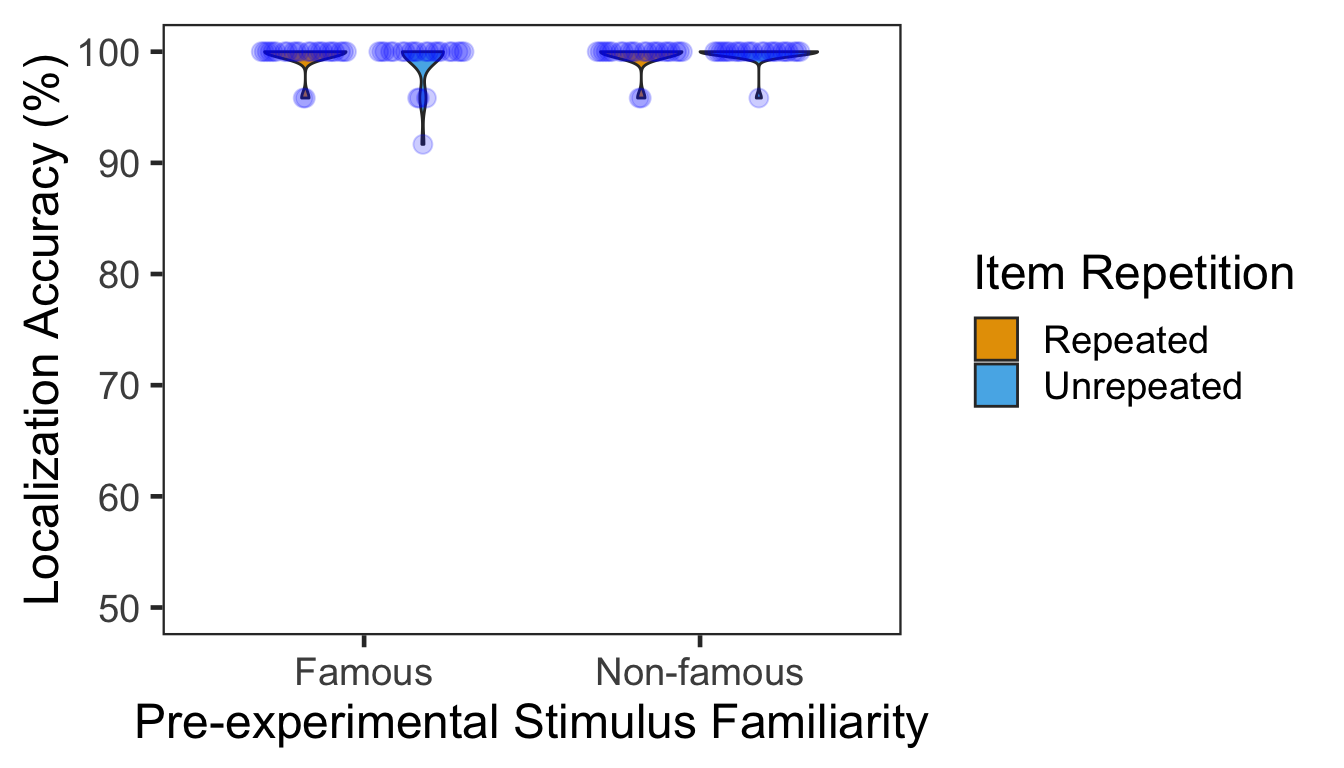### 2.1.1 ANOVA Mean percentage accuracy was submitted to a 2x2 mixed design ANOVA with pre-experimental stimulus familiarity as a between-participants factor and item repetition as a within-participant factor. No effects were significant. p2.aov <- aov_ez(id = "SID", dv = "Accuracy", data = P2slong, between = "Familiarity", within = "Repetition") anova(p2.aov, es = "pes") %>% kable(digits = 4) num Df den Df MSE F pes Pr(>F) Familiarity 1 46 1.8084 1.6000 0.0336 0.2123 Repetition 1 46 2.1859 0.3309 0.0071 0.5679 Familiarity:Repetition 1 46 2.1859 1.3237 0.0280 0.2559 # 3 Source Memory Test Phase In each trial, participants first indicated in which quadrant a given face appeared during the item-source association phase. Participants then rated how confident they were about their memory judgment. There were 48 trials in total. pre-experimental stimulus familiarity was a between-participants factor. Item repetition was a within-participant factor. P3 <- read.csv("data/data_FamSM_Exp4A_Face_TST.csv", header = T) P3$Familiarity = factor(P3$Familiarity, levels=c(1,2), labels=c("Famous","Non-famous")) P3$Repetition = factor(P3$Repetition, levels=c(1,2), labels=c("Repeated","Unrepeated")) glimpse(P3, width=70) ## Observations: 2,304 ## Variables: 10 ##$ SID         <int> 1, 1, 1, 1, 1, 1, 1, 1, 1, 1, 1, 1, 1, 1, 1, 1,…
## $Familiarity <fct> Non-famous, Non-famous, Non-famous, Non-famous,… ##$ Trial       <int> 1, 2, 3, 4, 5, 6, 7, 8, 9, 10, 11, 12, 13, 14, …
## $Repetition <fct> Unrepeated, Unrepeated, Repeated, Unrepeated, U… ##$ AscLoc      <int> 2, 4, 2, 1, 2, 1, 4, 4, 2, 1, 3, 4, 1, 2, 2, 4,…
## $SrcResp <int> 1, 1, 2, 2, 2, 1, 3, 1, 2, 1, 3, 3, 1, 1, 2, 1,… ##$ Corr        <int> 0, 0, 1, 0, 1, 1, 0, 0, 1, 1, 1, 0, 1, 0, 1, 0,…
## $RT <dbl> 6357.03, 5074.45, 2951.22, 1915.16, 1394.97, 48… ##$ Confident   <int> 1, 1, 1, 4, 4, 1, 4, 1, 4, 4, 1, 3, 1, 1, 4, 1,…
## $ImgName <fct> unknown_female03.jpg, unknown_male18.jpg, unkno… # 1. SID: participant ID # 2. Familiarity: pre-experimental familiarity. 1 = famous, 2 = non-famous # 3. Trial: 1~48 # 4. Repetition: 1 = repeated, 2 = unrepeated # 5. AscLoc: location (source) in which the item was presented in Phase 2; quadrants, 1~4 # 6. SrcResp: source response; quadrants, 1~4 # 7. Corr: correctness, 1=correct, 0=incorrect # 8. RT: reaction times in ms. # 9. Confident: confidence rating, 1~4 # 10. ImgName: name of stimuli table(P3$Familiarity, P3$SID) ## ## 1 2 3 4 5 6 7 8 9 10 11 12 13 14 15 16 17 18 19 20 ## Famous 0 48 0 48 0 48 0 48 0 48 0 48 0 48 0 48 0 48 0 48 ## Non-famous 48 0 48 0 48 0 48 0 48 0 48 0 48 0 48 0 48 0 48 0 ## ## 21 22 23 24 25 26 27 28 29 30 31 32 33 34 35 36 37 38 39 40 ## Famous 0 48 0 48 0 48 0 48 0 48 0 48 0 48 0 48 0 48 0 48 ## Non-famous 48 0 48 0 48 0 48 0 48 0 48 0 48 0 48 0 48 0 48 0 ## ## 41 42 43 44 45 46 47 48 ## Famous 0 48 0 48 0 48 0 48 ## Non-famous 48 0 48 0 48 0 48 0 table(P3$Repetition, P3$SID) ## ## 1 2 3 4 5 6 7 8 9 10 11 12 13 14 15 16 17 18 19 20 ## Repeated 24 24 24 24 24 24 24 24 24 24 24 24 24 24 24 24 24 24 24 24 ## Unrepeated 24 24 24 24 24 24 24 24 24 24 24 24 24 24 24 24 24 24 24 24 ## ## 21 22 23 24 25 26 27 28 29 30 31 32 33 34 35 36 37 38 39 40 ## Repeated 24 24 24 24 24 24 24 24 24 24 24 24 24 24 24 24 24 24 24 24 ## Unrepeated 24 24 24 24 24 24 24 24 24 24 24 24 24 24 24 24 24 24 24 24 ## ## 41 42 43 44 45 46 47 48 ## Repeated 24 24 24 24 24 24 24 24 ## Unrepeated 24 24 24 24 24 24 24 24 ## 3.1 Accuracy We calculated the mean and s.d. of individual participants’ mean percentage accuracy. In the following plot, red points and error bars represent the means and 95% within-participants CIs. # phase 3, subject-level, long-format P3ACCslong <- P3 %>% group_by(SID, Familiarity, Repetition) %>% summarise(Accuracy = mean(Corr)*100) %>% ungroup() # summary table P3ACCg <- P3ACCslong %>% group_by(Familiarity, Repetition) %>% summarise(M = mean(Accuracy), SD = sd(Accuracy)) %>% ungroup() P3ACCg %>% kable() Familiarity Repetition M SD Famous Repeated 56.59722 19.77060 Famous Unrepeated 70.13889 17.57775 Non-famous Repeated 57.46528 14.99694 Non-famous Unrepeated 45.65972 14.45879 # marginal means of famous vs. non-famous conditions. P3ACCslong %>% group_by(Familiarity) %>% summarise(M = mean(Accuracy), SD = sd(Accuracy)) %>% ungroup() %>% kable() Familiarity M SD Famous 63.36806 19.73072 Non-famous 51.56250 15.74643 # marginal means of repeated vs. unrepeated conditions. P3ACCslong %>% group_by(Repetition) %>% summarise(M = mean(Accuracy), SD = sd(Accuracy)) %>% ungroup() %>% kable() Repetition M SD Repeated 57.03125 17.36473 Unrepeated 57.89931 20.16187 # wide format, needed for geom_segments. P3ACCswide <- P3ACCslong %>% spread(key = Repetition, value = Accuracy) # group level, needed for printing & geom_pointrange # Rmisc must be called indirectly due to incompatibility between plyr and dplyr. P3ACCg$ci <- Rmisc::summarySEwithin(data = P3ACCslong, measurevar = "Accuracy", idvar = "SID",
withinvars = "Repetition", betweenvars = "Familiarity")$ci P3ACCg$Accuracy <- P3ACCg$M ggplot(data=P3ACCslong, aes(x=Familiarity, y=Accuracy, fill=Repetition)) + geom_violin(width = 0.5, trim=TRUE) + geom_point(position=position_dodge(0.5), color="gray80", size=1.8, show.legend = FALSE) + geom_segment(data=filter(P3ACCswide, Familiarity=="Famous"), inherit.aes = FALSE, aes(x=1-.12, y=filter(P3ACCswide, Familiarity=="Famous")$Repeated,
xend=1+.12, yend=filter(P3ACCswide, Familiarity=="Famous")$Unrepeated), color="gray80") + geom_segment(data=filter(P3ACCswide, Familiarity=="Non-famous"), inherit.aes = FALSE, aes(x=2-.12, y=filter(P3ACCswide, Familiarity=="Non-famous")$Repeated,
xend=2+.12, yend=filter(P3ACCswide, Familiarity=="Non-famous")$Unrepeated), color="gray80") + geom_pointrange(data=P3ACCg, aes(x = Familiarity, ymin = Accuracy-ci, ymax = Accuracy+ci, group = Repetition), position = position_dodge(0.5), color = "darkred", size = 1, show.legend = FALSE) + scale_fill_manual(values=c("#E69F00", "#56B4E9"), labels=c("Repeated", "Unrepeated")) + labs(x = "Pre-experimental Stimulus Familiarity", y = "Source Memory Accuracy (%)", fill='Item Repetition') + coord_cartesian(ylim = c(0, 100), clip = "on") + theme_bw(base_size = 18) + theme(panel.grid.major = element_blank(), panel.grid.minor = element_blank())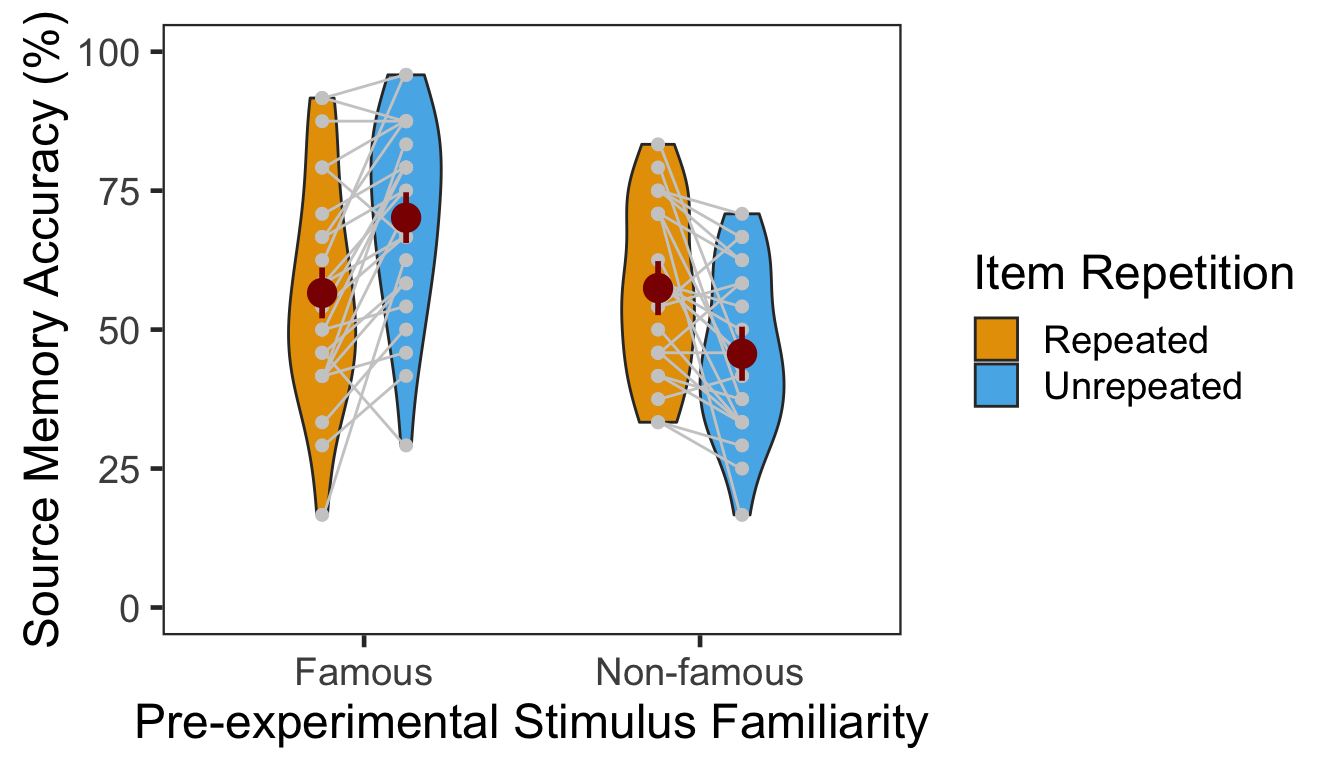The effect of item repetition was modulated by the pre-experimental stimulus familiarity of the items. For famous faces, the locations of unrepeated items were better remembered (novelty benefit). For non-famous faces, the locations of repeated items were better remembered (familiarity benefit). These crossed effects were on top of the general familiarity benefit, in which source memory was more accurate for famous faces than for non-famous faces. ### 3.1.1 ANOVA Individuals’ mean percentage accuracy was submitted to a 2x2 mixed design ANOVA with pre-experimental stimulus familiarity as a between-participants factor (famous vs. non-famous) and item repetition as a within-participant factor (Repeated vs. Unrepeated). p3.corr.aov <- aov_ez(id = "SID", dv = "Accuracy", data = P3ACCslong, between = "Familiarity", within = "Repetition") anova(p3.corr.aov, es = "pes") %>% kable(digits = 4) num Df den Df MSE F pes Pr(>F) Familiarity 1 46 442.0164 7.5674 0.1413 0.0085 Repetition 1 46 124.8931 0.1448 0.0031 0.7053 Familiarity:Repetition 1 46 124.8931 30.8655 0.4016 0.0000 The main effect of pre-experimental stimulus familiarity and the two-way interaction were both significant. Additionally, we performed two separate one-way repeated-measures ANOVAs as post-hoc analyses. The table below shows the effect of item repetition for famous faces. ci95 <- P3ACCswide %>% filter(Familiarity=="Famous") %>% mutate(Diff = Unrepeated - Repeated) %>% summarise(lower = mean(Diff) - qt(0.975,df=n()-1)*sd(Diff)/sqrt(n()), upper = mean(Diff) + qt(0.975,df=n()-1)*sd(Diff)/sqrt(n())) p3.corr.aov.r1 <- aov_ez(id = "SID", dv = "Accuracy", within = "Repetition", data = filter(P3ACCslong, Familiarity == "Famous")) anova(p3.corr.aov.r1, es = "pes") %>% kable(digits = 4) num Df den Df MSE F pes Pr(>F) Repetition 1 23 117.1875 18.7778 0.4495 2e-04 In the famous face group, source memory was more accurate for unrepeated than repeated faces. The 95% CI of difference between the means was [7.08, 20.01]. The table below shows the effect of item repetition for non-famous faces. ci95 <- P3ACCswide %>% filter(Familiarity=="Non-famous") %>% mutate(Diff = Repeated - Unrepeated) %>% summarise(lower = mean(Diff) - qt(0.975,df=n()-1)*sd(Diff)/sqrt(n()), upper = mean(Diff) + qt(0.975,df=n()-1)*sd(Diff)/sqrt(n())) p3.corr.aov.r2 <- aov_ez(id = "SID", dv = "Accuracy", within = "Repetition", data = filter(P3ACCslong, Familiarity == "Non-famous")) anova(p3.corr.aov.r2, es = "pes") %>% kable(digits = 4) num Df den Df MSE F pes Pr(>F) Repetition 1 23 132.5986 12.6129 0.3542 0.0017 In the non-famous face group, source memory was more accurate for repeated than unrepeated faces. The 95% CI of difference between the means was [4.93, 18.68]. ### 3.1.2 GLMM To supplement conventional ANOVAs, we tested GLMMs on source memory accuracy. This mixed modeling approach with a binomial link function is expected to properly handle binary data such as source memory responses (i.e., correct or not; Jaeger, 2008). We built the full model (full1) with two fixed effects (pre-experimental stimulus familiarity and item repetition) and their interaction. The model also included maximal random effects structure (Barr, Levy, Scheepers, & Tily, 2013): both by-participant and by-item random intercepts, and by-participant random slopes for item repetition. In case the maximal model does not converge successfully, we built another model (full2) with the maximal random structure but with the correlations among the random terms removed (Singmann, 2018). To fit the models, we used the mixed() of the afex package (Singmann, Bolker, & Westfall, 2017) which was built on the lmer() of the lme4 package (Bates, Maechler, Bolker, & Walker, 2015). The mixed() assessed the statistical significance of fixed effects by comparing a model with the effect in question against its nested model which lacked the effect in question. P-values of the effects were obtained by likelihood ratio tests (LRT). (nc <- detectCores()) cl <- makeCluster(rep("localhost", nc)) full1 <- afex::mixed(Corr ~ Familiarity*Repetition + (Repetition|SID) + (1|ImgName), P3, method = "LRT", cl = cl, family=binomial(link="logit"), control = glmerControl(optCtrl = list(maxfun = 1e6))) full2 <- afex::mixed(Corr ~ Familiarity*Repetition + (Repetition||SID) + (1|ImgName), P3, method = "LRT", cl = cl, family=binomial(link="logit"), control = glmerControl(optCtrl = list(maxfun = 1e6)), expand_re = TRUE) stopCluster(cl) The table below presents the LRT results of the models full1 and full2. full.compare <- cbind(afex::nice(full1), afex::nice(full2)[,-c(1,2)]) colnames(full.compare)[c(3,4,5,6)] <- c("full1 Chisq", "p", "full2 Chisq", "p") full.compare %>% kable() Effect df full1 Chisq p full2 Chisq p Familiarity 1 7.22 ** .007 7.25 ** .007 Repetition 1 0.47 .49 0.51 .47 Familiarity:Repetition 1 25.11 *** <.0001 25.53 *** <.0001 The p-values from the two models were highly similar to each other. Post-hoc analysis results are summarized in the table below. The results from the pairwise comparisons were consistent with those from the ANOVA. Item repetition impaired source memory for famous faces whereas it improved source memory for non-famous faces. emmeans(full1, pairwise ~ Repetition | Familiarity, type = "response")$contrasts %>% kable()
contrast Familiarity odds.ratio SE df z.ratio p.value
Repeated / Unrepeated Famous 0.5200752 0.0772337 Inf -4.402426 0.0000107
Repeated / Unrepeated Non-famous 1.6703859 0.2330347 Inf 3.677560 0.0002355

## 3.2 Confidence

The table below presents the mean and s.d. of individual participants’ confidence ratings in each condition. The pattern of confidence ratings was qualitatively identical to that of source memory accuracy; we observed the novelty benefit for famous faces and the familiarity benefit for non-famous faces. In the following plot, red points and error bars represent the means and 95% within-participants CIs.

P3CFslong <- P3 %>% group_by(SID, Familiarity, Repetition) %>%
summarise(Confidence = mean(Confident)) %>%
ungroup()

P3CFg <- P3CFslong %>%
group_by(Familiarity, Repetition) %>%
summarise(M = mean(Confidence), SD = sd(Confidence)) %>%
ungroup()
P3CFg %>% kable()
Familiarity Repetition M SD
Famous Repeated 2.689236 0.4577531
Famous Unrepeated 2.942708 0.5883342
Non-famous Repeated 2.807292 0.5439351
Non-famous Unrepeated 2.133681 0.4987877
# marginal means of famous vs. non-famous conditions.
P3CFslong %>% group_by(Familiarity) %>%
summarise(M = mean(Confidence), SD = sd(Confidence)) %>%
ungroup() %>% kable()
Familiarity M SD
Famous 2.815972 0.5369636
Non-famous 2.470486 0.6183726
# marginal means of repeated vs. unrepeated conditions.
P3CFslong %>% group_by(Repetition) %>%
summarise(M = mean(Confidence), SD = sd(Confidence)) %>%
ungroup() %>% kable()
Repetition M SD
Repeated 2.748264 0.5008827
Unrepeated 2.538194 0.6769395
# wide format, needed for geom_segments.
P3CFswide <- P3CFslong %>% spread(key = Repetition, value = Confidence)

# group level, needed for printing & geom_pointrange
# Rmisc must be called indirectly due to incompatibility between plyr and dplyr.
P3CFg$ci <- Rmisc::summarySEwithin(data = P3CFslong, measurevar = "Confidence", idvar = "SID", withinvars = "Repetition", betweenvars = "Familiarity")$ci
P3CFg$Confidence <- P3CFg$M

ggplot(data=P3CFslong, aes(x=Familiarity, y=Confidence, fill=Repetition)) +
geom_violin(width = 0.5, trim=TRUE) +
geom_point(position=position_dodge(0.5), color="gray80", size=1.8, show.legend = FALSE) +
geom_segment(data=filter(P3CFswide, Familiarity=="Famous"), inherit.aes = FALSE,
aes(x=1-.12, y=filter(P3CFswide, Familiarity=="Famous")$Repeated, xend=1+.12, yend=filter(P3CFswide, Familiarity=="Famous")$Unrepeated),
color="gray80") +
geom_segment(data=filter(P3CFswide, Familiarity=="Non-famous"), inherit.aes = FALSE,
aes(x=2-.12, y=filter(P3CFswide, Familiarity=="Non-famous")$Repeated, xend=2+.12, yend=filter(P3CFswide, Familiarity=="Non-famous")$Unrepeated),
color="gray80") +
geom_pointrange(data=P3CFg,
aes(x = Familiarity, ymin = Confidence-ci, ymax = Confidence+ci, group = Repetition),
position = position_dodge(0.5), color = "darkred", size = 1, show.legend = FALSE) +
scale_fill_manual(values=c("#E69F00", "#56B4E9"),
labels=c("Repeated", "Unrepeated")) +
labs(x = "Pre-experimental Stimulus Familiarity",
y = "Source Memory Confidence",
fill='Item Repetition') +
coord_cartesian(ylim = c(1, 4), clip = "on") +
theme_bw(base_size = 18) +
theme(panel.grid.major = element_blank(),
panel.grid.minor = element_blank())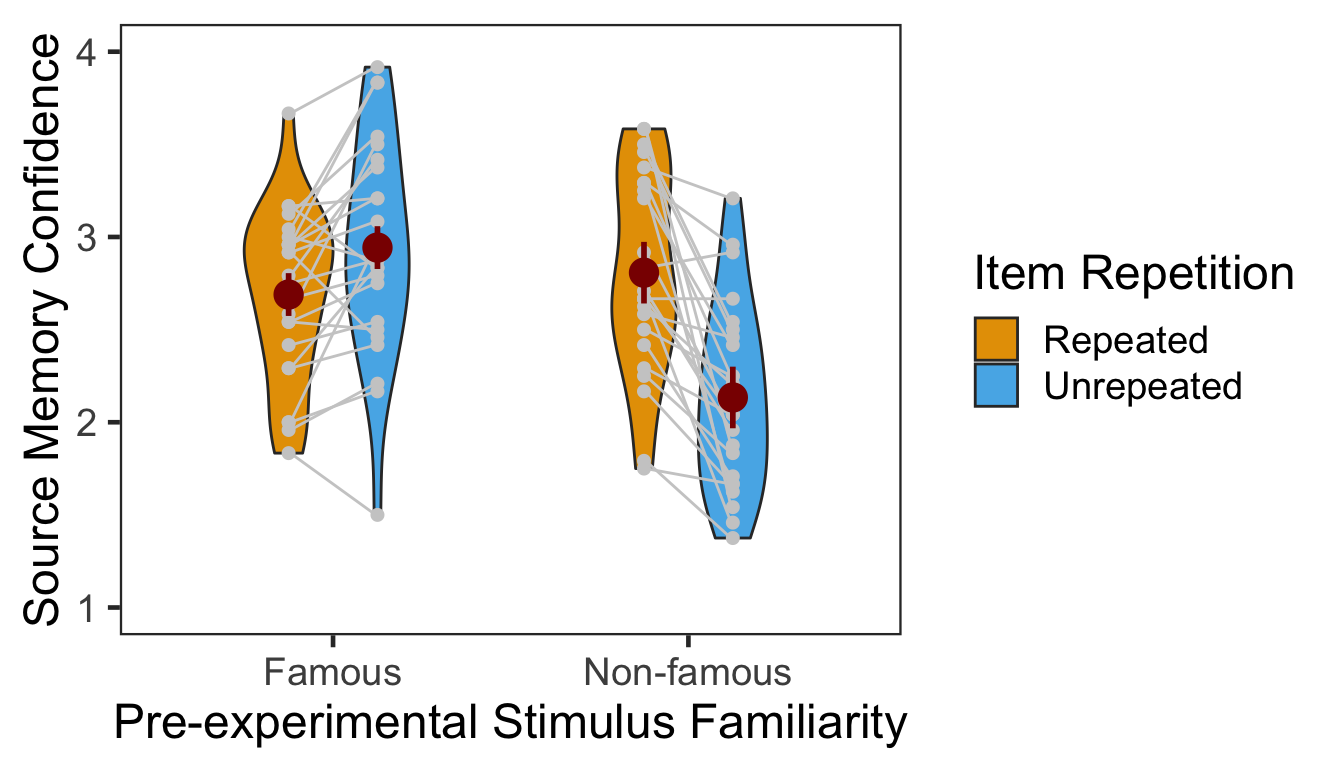### 3.2.1 ANOVA

Individuals’ confidence ratings were submitted to a 2x2 mixed design ANOVA with pre-experimental stimulus familiarity as a between-participants factor and item repetition as a within-participant factor.

p3.conf.aov <- aov_ez(id = "SID", dv = "Confidence", data = P3CFslong,
between = "Familiarity", within = "Repetition")
anova(p3.conf.aov, es = "pes") %>% kable(digits = 4)
num Df den Df MSE F pes Pr(>F)
Familiarity 1 46 0.4353 6.5815 0.1252 0.0136
Repetition 1 46 0.1149 9.2170 0.1669 0.0039
Familiarity:Repetition 1 46 0.1149 44.8788 0.4938 0.0000

The two-way interaction as well as both main effects reached significance. Next we performed two additional one-way repeated-measures ANOVAs as post-hoc analyses. The first table below presents the effect of item repetition for famous faces, and the second presents the same effect for non-famous faces.

ci95 <- P3CFswide %>% filter(Familiarity=="Famous") %>%
mutate(Diff = Unrepeated - Repeated) %>%
summarise(lower = mean(Diff) - qt(0.975,df=n()-1)*sd(Diff)/sqrt(n()),
upper = mean(Diff) + qt(0.975,df=n()-1)*sd(Diff)/sqrt(n()))

p3.conf.aov.r1 <- aov_ez(id = "SID", dv = "Confidence", within = "Repetition",
data = filter(P3CFslong, Familiarity == "Famous"))
anova(p3.conf.aov.r1, es = "pes") %>% kable(digits = 4)
num Df den Df MSE F pes Pr(>F)
Repetition 1 23 0.0748 10.3075 0.3095 0.0039

In the famous face group, confidence ratings were higher for unrepeated than repeated faces. The 95% CI of difference between the means was [0.09, 0.42].

ci95 <- P3CFswide %>% filter(Familiarity=="Non-famous") %>%
mutate(Diff = Repeated - Unrepeated) %>%
summarise(lower = mean(Diff) - qt(0.975,df=n()-1)*sd(Diff)/sqrt(n()),
upper = mean(Diff) + qt(0.975,df=n()-1)*sd(Diff)/sqrt(n()))

p3.conf.aov.r2 <- aov_ez(id = "SID", dv = "Confidence", within = "Repetition",
data = filter(P3CFslong, Familiarity == "Non-famous"))
anova(p3.conf.aov.r2, es = "pes") %>% kable(digits = 4)
num Df den Df MSE F pes Pr(>F)
Repetition 1 23 0.155 35.1253 0.6043 0

In the non-famous face group, confidence ratings were higher for repeated than unrepeated faces. The 95% CI of difference between the means was [0.44, 0.91].

### 3.2.2 CLMM

The responses from a Likert-type scale are ordinal. Especially for the rating items with numerical response formats containing four or fewer categories, it is recommended to use categorical data analysis approaches, rather than treating the responses as continuous data (Harpe, 2015).

Here we employed the cumulative link mixed modeling using the clmm() of the package ordinal (Christensen, submitted). The specification of the full model was the same as the mixed() above. To determine the statistical significance, the LRT compared models with or without the fixed effect of interest.

P3R <- P3
P3R$Confident = factor(P3R$Confident, ordered = TRUE)
P3R$SID = factor(P3R$SID)

cm.full <- clmm(Confident ~ Familiarity * Repetition + (Repetition|SID) + (1|ImgName), data=P3R)
cm.red1 <- clmm(Confident ~ Familiarity + Repetition + (Repetition|SID) + (1|ImgName), data=P3R)
cm.red2 <- clmm(Confident ~ Repetition + (Repetition|SID) + (1|ImgName), data=P3R)
cm.red3 <- clmm(Confident ~ 1 + (Repetition|SID) + (1|ImgName), data=P3R) 
cm.comp <- anova(cm.full, cm.red1, cm.red2, cm.red3)

data.frame(Effect = c("Familiarity", "Repetition", "Familiarity:Repetition"),
df = 1, Chisq = cm.comp$LR.stat[2:4], p = cm.comp$Pr(>Chisq)[2:4]) %>% kable()
Effect df Chisq p
Familiarity 1 3.4316676 0.0639575
Repetition 1 0.3860451 0.5343856
Familiarity:Repetition 1 32.7947040 0.0000000

The LRT revealed a significant two-way interaction. No main effects were significant. Next we performed pairwise comparisons as post-hoc analyses. As shown in the following table, the results of the pairwise comparisons were consistent with those from the ANOVA approach.

emmeans(cm.full, pairwise ~ Repetition | Familiarity)$contrasts %>% kable() contrast Familiarity estimate SE df z.ratio p.value Repeated - Unrepeated Famous -0.5496718 0.1881596 Inf -2.921305 0.0034857 Repeated - Unrepeated Non-famous 1.2514894 0.1861433 Inf 6.723259 0.0000000 Below is the plot of estimated marginal means, which were extracted from the fitted CLMM. The estimated distribution of confidence ratings shows the interaction between item repetition and pre-experimental stimulus familiarity. temp <- emmeans(cm.full,~Familiarity:Repetition|cut, mode="linear.predictor") temp <- rating.emmeans(temp) temp <- temp %>% unite(Condition, c("Familiarity", "Repetition")) ggplot(data = temp, aes(x = Rating, y = Prob, group = Condition)) + geom_line(aes(color = Condition), size = 1.2) + geom_point(aes(shape = Condition, color = Condition), size = 4, fill = "white", stroke = 1.2) + scale_color_manual(values=c("#E69F00", "#E69F00", "#56B4E9", "#56B4E9")) + scale_shape_manual(name="Condition", values=c(21,24,21,24)) + labs(y = "Response Probability", x = "Rating") + expand_limits(y=0) + scale_y_continuous(limits = c(0, 0.5)) + scale_x_discrete(labels = c("1" = "1(Guessed)","4"="4(Sure)")) + theme_minimal() + theme(text = element_text(size=18))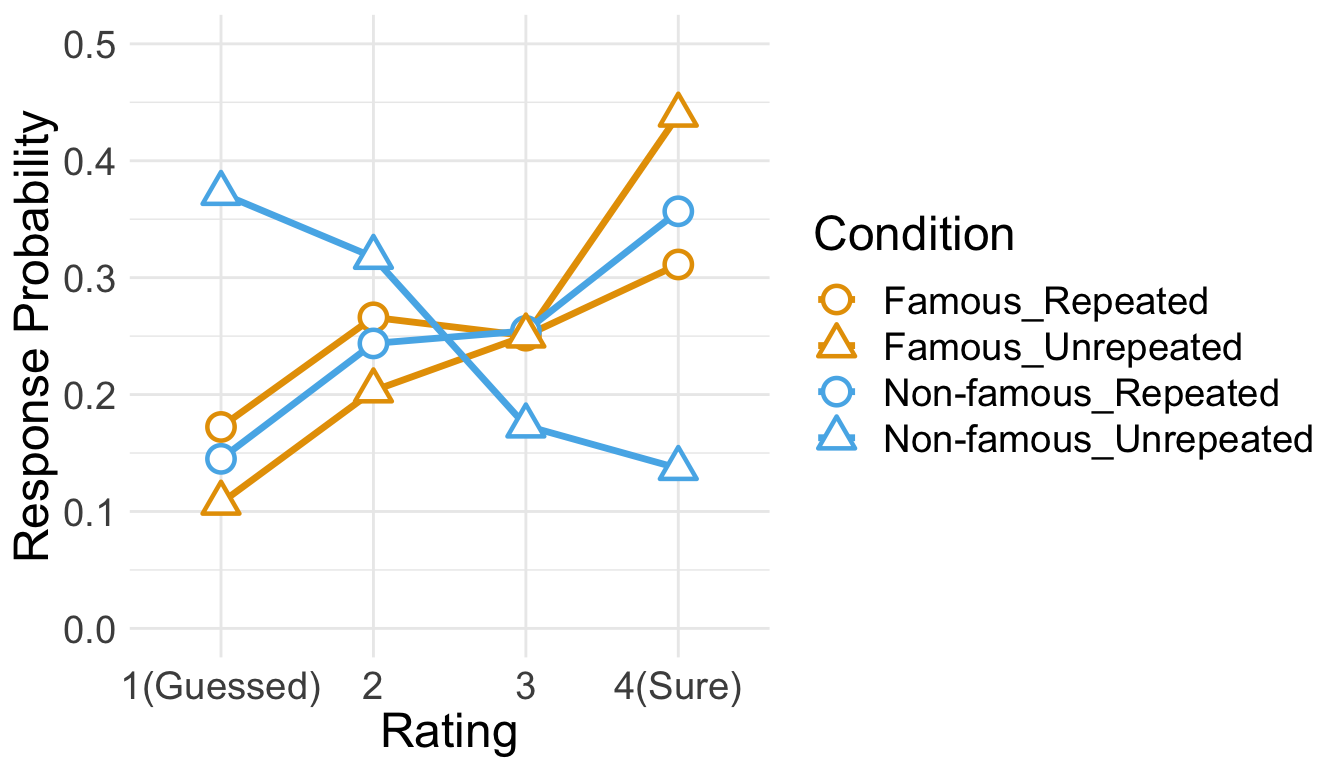## 3.3 RT Only RTs from correct trials were analyzed. Before analysis, we first removed RTs either shorter than 200ms or longer than 10s. Then, from the RT distribution of each participant, RTs beyond 3 s.d. from the mean were additionally removed. cP3 <- P3 %>% filter(Corr==1) sP3 <- cP3 %>% filter(RT > 200 & RT < 10000) %>% group_by(SID) %>% nest() %>% mutate(lbound = map(data, ~mean(.$RT)-3*sd(.$RT)), ubound = map(data, ~mean(.$RT)+3*sd(.$RT))) %>% unnest(lbound, ubound) %>% unnest(data) %>% ungroup() %>% mutate(Outlier = (RT < lbound)|(RT > ubound)) %>% filter(Outlier == FALSE) %>% select(SID, Familiarity, Repetition, RT, ImgName) 100 - 100*nrow(sP3)/nrow(cP3) ##  5.362538 This trimming procedure removed 5.36% of correct trials. Since the overall source memory accuracy was not high, only small numbers of correct trials were available after trimming. The following table summarizes the numbers of RTs submitted to subsequent analyses. No participant had more than 25 trials per condition. sP3 %>% group_by(SID, Familiarity, Repetition) %>% summarise(NumTrial = length(RT)) %>% ungroup() %>% group_by(Familiarity, Repetition) %>% summarise(Mean = mean(NumTrial), Median = median(NumTrial), Min = min(NumTrial), Max = max(NumTrial)) %>% ungroup %>% kable() Familiarity Repetition Mean Median Min Max Famous Repeated 12.83333 12.5 4 21 Famous Unrepeated 16.37500 17.0 7 23 Non-famous Repeated 12.95833 13.0 8 19 Non-famous Unrepeated 10.04167 10.0 3 16 den1 <- ggplot(cP3, aes(x=RT)) + geom_density() + theme_bw(base_size = 18) + theme(panel.grid.major = element_blank(), panel.grid.minor = element_blank(), axis.text.y = element_blank(), axis.ticks.y = element_blank()) den2 <- ggplot(sP3, aes(x=RT)) + geom_density() + theme_bw(base_size = 18) + labs(x = "Trimmed RT") + theme(panel.grid.major = element_blank(), panel.grid.minor = element_blank(), axis.text.y = element_blank(), axis.ticks.y = element_blank()) den1 + den2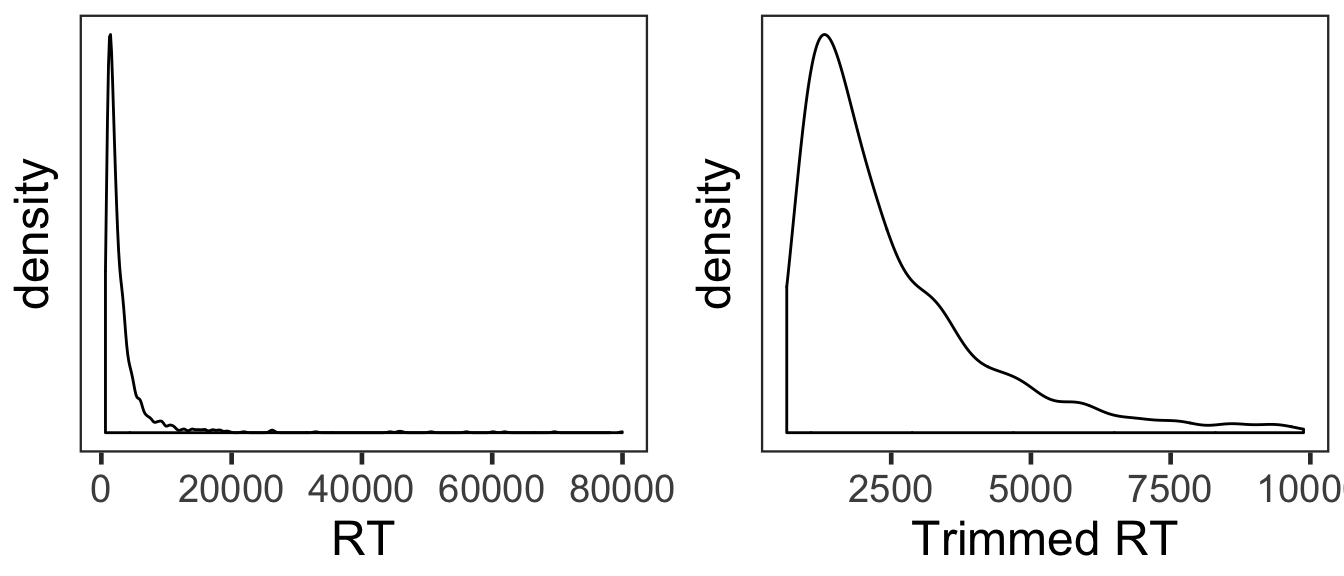The overall RT distribution was highly skewed even after trimming. Given limited numbers of RTs and its skewed distribution, any results from the current RT analyses should be interpreted with caution and preferably corroborated with other measures. We calculated the mean and s.d. of individual participants’ mean RTs. The overall pattern of RTs across the conditions was consistent with that of source memory accuracy and confidence ratings. In the following plot, red points and error bars represent the means and 95% within-participants CIs. P3RTslong <- sP3 %>% group_by(SID, Familiarity, Repetition) %>% summarise(RT = mean(RT)) %>% ungroup() P3RTg <- P3RTslong %>% group_by(Familiarity, Repetition) %>% summarise(M = mean(RT), SD = sd(RT)) %>% ungroup() P3RTg %>% kable() Familiarity Repetition M SD Famous Repeated 2389.634 710.8305 Famous Unrepeated 1943.214 670.0161 Non-famous Repeated 2994.780 946.9946 Non-famous Unrepeated 3177.818 927.3634 # wide format, needed for geom_segments. P3RTswide <- P3RTslong %>% spread(key = Repetition, value = RT) # group level, needed for printing & geom_pointrange # Rmisc must be called indirectly due to incompatibility between plyr and dplyr. P3RTg$ci <- Rmisc::summarySEwithin(data = P3RTslong, measurevar = "RT", idvar = "SID",
withinvars = "Repetition", betweenvars = "Familiarity")$ci P3RTg$RT <- P3RTg$M ggplot(data=P3RTslong, aes(x=Familiarity, y=RT, fill=Repetition)) + geom_violin(width = 0.5, trim=TRUE) + geom_point(position=position_dodge(0.5), color="gray80", size=1.8, show.legend = FALSE) + geom_segment(data=filter(P3RTswide, Familiarity=="Famous"), inherit.aes = FALSE, aes(x=1-.12, y=filter(P3RTswide, Familiarity=="Famous")$Repeated,
xend=1+.12, yend=filter(P3RTswide, Familiarity=="Famous")$Unrepeated), color="gray80") + geom_segment(data=filter(P3RTswide, Familiarity=="Non-famous"), inherit.aes = FALSE, aes(x=2-.12, y=filter(P3RTswide, Familiarity=="Non-famous")$Repeated,
xend=2+.12, yend=filter(P3RTswide, Familiarity=="Non-famous")$Unrepeated), color="gray80") + geom_pointrange(data=P3RTg, aes(x = Familiarity, ymin = RT-ci, ymax = RT+ci, group = Repetition), position = position_dodge(0.5), color = "darkred", size = 1, show.legend = FALSE) + scale_fill_manual(values=c("#E69F00", "#56B4E9"), labels=c("Repeated", "Unrepeated")) + labs(x = "Pre-experimental Stimulus Familiarity", y = "Response Times (ms)", fill='Item Repetition') + theme_bw(base_size = 18) + theme(panel.grid.major = element_blank(), panel.grid.minor = element_blank())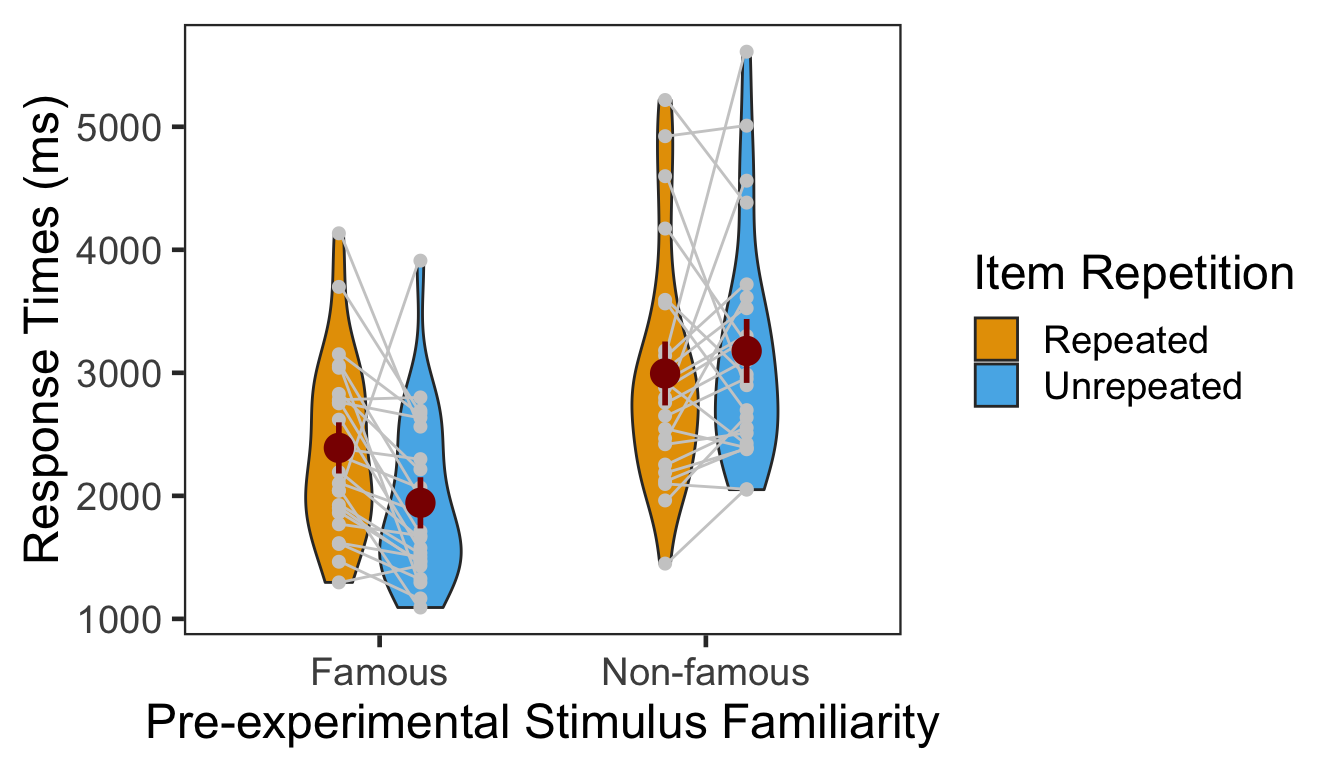### 3.3.1 ANOVA Individuals’ mean RTs were submitted to a 2x2 mixed design ANOVA with pre-experimental stimulus familiarity as a between-participants factor and item repetition as a within-participant factor. p3.rt.aov <- aov_ez(id = "SID", dv = "RT", data = sP3, between = "Familiarity", within = "Repetition") anova(p3.rt.aov, es = "pes") %>% kable(digits = 4) num Df den Df MSE F pes Pr(>F) Familiarity 1 46 1044889.5 19.4356 0.2970 0.0001 Repetition 1 46 310612.1 1.3400 0.0283 0.2530 Familiarity:Repetition 1 46 310612.1 7.6536 0.1426 0.0081 The main effect of pre-experimental stimulus familiarity and the two-way interaction were both significant. We then performed two one-way repeated-measures ANOVAs as post-hoc analyses. The first table below presents the effect of item repetition for famous faces, and the second table presents the same effect for non-famous faces. p3.rt.aov.r1 <- aov_ez(id = "SID", dv = "RT", within = "Repetition", data = filter(sP3, Familiarity == "Famous")) anova(p3.rt.aov.r1, es = "pes") %>% kable(digits = 4) num Df den Df MSE F pes Pr(>F) Repetition 1 23 242799.6 9.8496 0.2998 0.0046 The effect of item repetition was significant in the famous condition. p3.rt.aov.r2 <- aov_ez(id = "SID", dv = "RT", within = "Repetition", data = filter(sP3, Familiarity == "Non-famous")) anova(p3.rt.aov.r2, es = "pes") %>% kable(digits = 4) num Df den Df MSE F pes Pr(>F) Repetition 1 23 378424.6 1.0624 0.0442 0.3134 The effect of item repetition was not significant in the non-famous condition. ### 3.3.2 GLMM To supplement conventional ANOVAs, we performed GLMMs on source memory RT. We built the full model (rt.full) that assumes an inverse Gaussian distribution and a linear relationship (identity link function) between the predictors and RT (Lo & Andrews, 2015). The model included two fixed effects (pre-experimental stimulus familiarity and item repetition) as well as their interaction. The model also included maximal random effects structure (Barr, Levy, Scheepers, & Tily, 2013): both by-participant and by-item random intercepts, and by-participant random slopes for item repetition. This approach is expected to properly handle unbalanced data with a small sample size and skewed distribution such as our RT data.2 (nc <- detectCores()) cl <- makeCluster(rep("localhost", nc)) rt.full <- afex::mixed(RT ~ Familiarity*Repetition + (Repetition|SID) + (1|ImgName), sP3, method = "LRT", cl = cl, family=inverse.gaussian(link="identity"), control = glmerControl(optCtrl = list(maxfun = 1e6))) stopCluster(cl) anova(rt.full) %>% kable() Df Chisq Chi Df Pr(>Chisq) Familiarity 8 2.187735 1 0.1391138 Repetition 8 1.327305 1 0.2492852 Familiarity:Repetition 8 4.134498 1 0.0420175 The two-way interaction was significant. Neither main effects were significant. In subsequent pairwise comparisons, the effect of item repetition was significant only for famous faces (see below). emmeans(rt.full, pairwise ~ Repetition | Familiarity, type = "response")$contrasts %>% kable()
contrast Familiarity estimate SE df z.ratio p.value
Repeated - Unrepeated Famous 453.38475 109.2457 Inf 4.1501379 0.0000332
Repeated - Unrepeated Non-famous -75.94242 111.3944 Inf -0.6817437 0.4954011

# 4 Session Info

sessionInfo()
## R version 3.5.3 (2019-03-11)
## Platform: x86_64-apple-darwin15.6.0 (64-bit)
## Running under: macOS Mojave 10.14.4
##
## Matrix products: default
## BLAS: /Library/Frameworks/R.framework/Versions/3.5/Resources/lib/libRblas.0.dylib
## LAPACK: /Library/Frameworks/R.framework/Versions/3.5/Resources/lib/libRlapack.dylib
##
## locale:
##  en_US.UTF-8/en_US.UTF-8/en_US.UTF-8/C/en_US.UTF-8/en_US.UTF-8
##
## attached base packages:
##  parallel  stats     graphics  grDevices utils     datasets  methods
##  base
##
## other attached packages:
##   klippy_0.0.0.9500    patchwork_0.0.1      RVAideMemoire_0.9-73
##   ggbeeswarm_0.6.0     ordinal_2019.3-9     emmeans_1.3.3
##   afex_0.23-0          lme4_1.1-21          Matrix_1.2-17
##  car_3.0-2            carData_3.0-2        knitr_1.22
##  forcats_0.4.0        stringr_1.4.0        dplyr_0.8.0.1
##  tibble_2.1.1         ggplot2_3.1.0        tidyverse_1.2.1
##  Rmisc_1.5            plyr_1.8.4           lattice_0.20-38
##  pacman_0.5.1
##
## loaded via a namespace (and not attached):
##   nlme_3.1-137      lubridate_1.7.4   httr_1.4.0
##   numDeriv_2016.8-1 tools_3.5.3       backports_1.1.3
##   utf8_1.1.4        R6_2.4.0          vipor_0.4.5
##  lazyeval_0.2.2    colorspace_1.4-1  withr_2.1.2
##  tidyselect_0.2.5  curl_3.3          compiler_3.5.3
##  cli_1.1.0         rvest_0.3.2       xml2_1.2.0
##  sandwich_2.5-0    labeling_0.3      scales_1.0.0
##  mvtnorm_1.0-10    digest_0.6.18     foreign_0.8-71
##  minqa_1.2.4       rmarkdown_1.12    rio_0.5.16
##  pkgconfig_2.0.2   htmltools_0.3.6   highr_0.8
##  generics_0.0.2    zoo_1.8-5         jsonlite_1.6
##  zip_2.0.1         magrittr_1.5      fansi_0.4.0
##  Rcpp_1.0.1        munsell_0.5.0     abind_1.4-5
##  ucminf_1.1-4      stringi_1.4.3     multcomp_1.4-10
##  yaml_2.2.0        MASS_7.3-51.3     grid_3.5.3
##  crayon_1.3.4      haven_2.1.0       splines_3.5.3
##  hms_0.4.2         pillar_1.3.1      boot_1.3-20
##  estimability_1.3  reshape2_1.4.3    codetools_0.2-16
##  glue_1.3.1        evaluate_0.13     data.table_1.12.0
##  modelr_0.1.4      nloptr_1.2.1      cellranger_1.1.0
##  gtable_0.3.0      assertthat_0.2.1  xfun_0.5
##  openxlsx_4.1.0    xtable_1.8-3      broom_0.5.1
##  coda_0.19-2       survival_2.44-1   lmerTest_3.1-0
##  beeswarm_0.2.3    TH.data_1.0-10

1. One additional participant in the non-famous face group was excluded due to failing to respond in two thirds of trials in the second phase.

2. We tested additional GLMMs on source memory RTs. One model assumed a Gamma distribution and a linear relationship (identity link function) between the predictors and RTs. The other models adopted non-linear transformations of RTs (such as -1000/RT or log(RT)). None of the models, however, converged onto a stable solution.